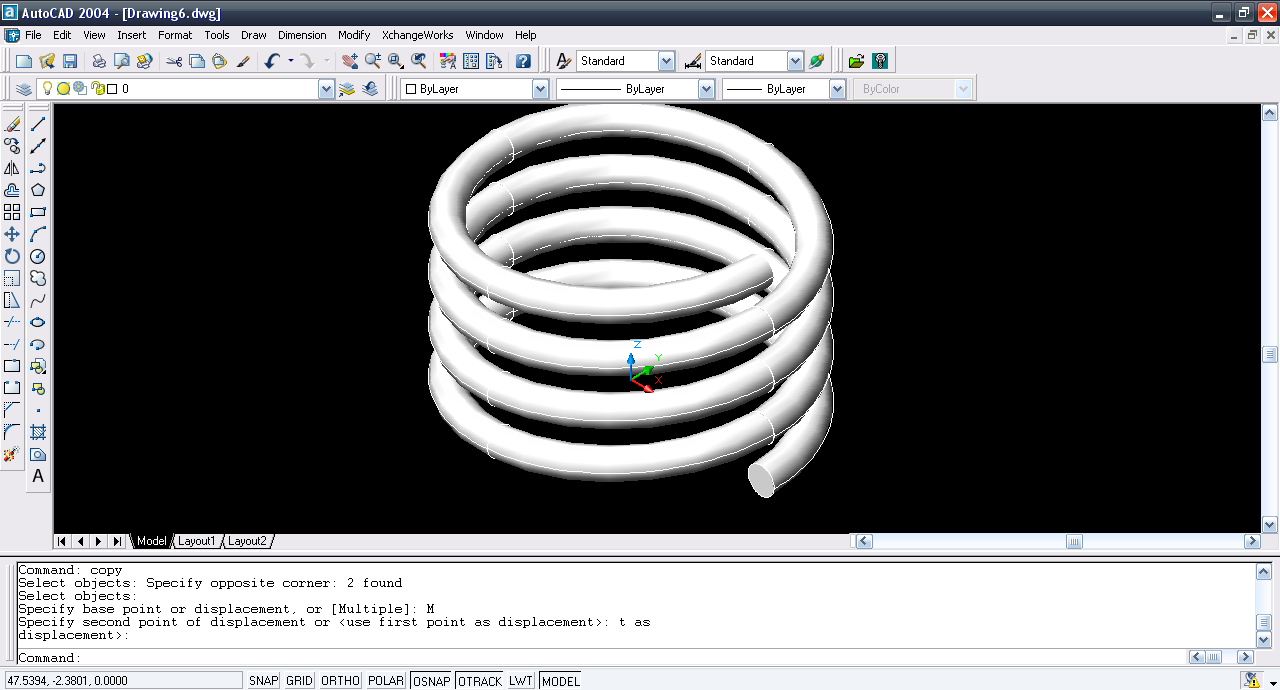# Tutorial - Modeling a spring in AutoCAD using slice tool?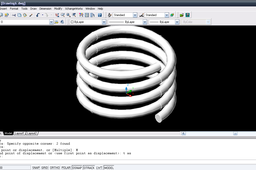Here is the tutorial.

1. ### Step 1:

Start AutoCAD and input Torus command.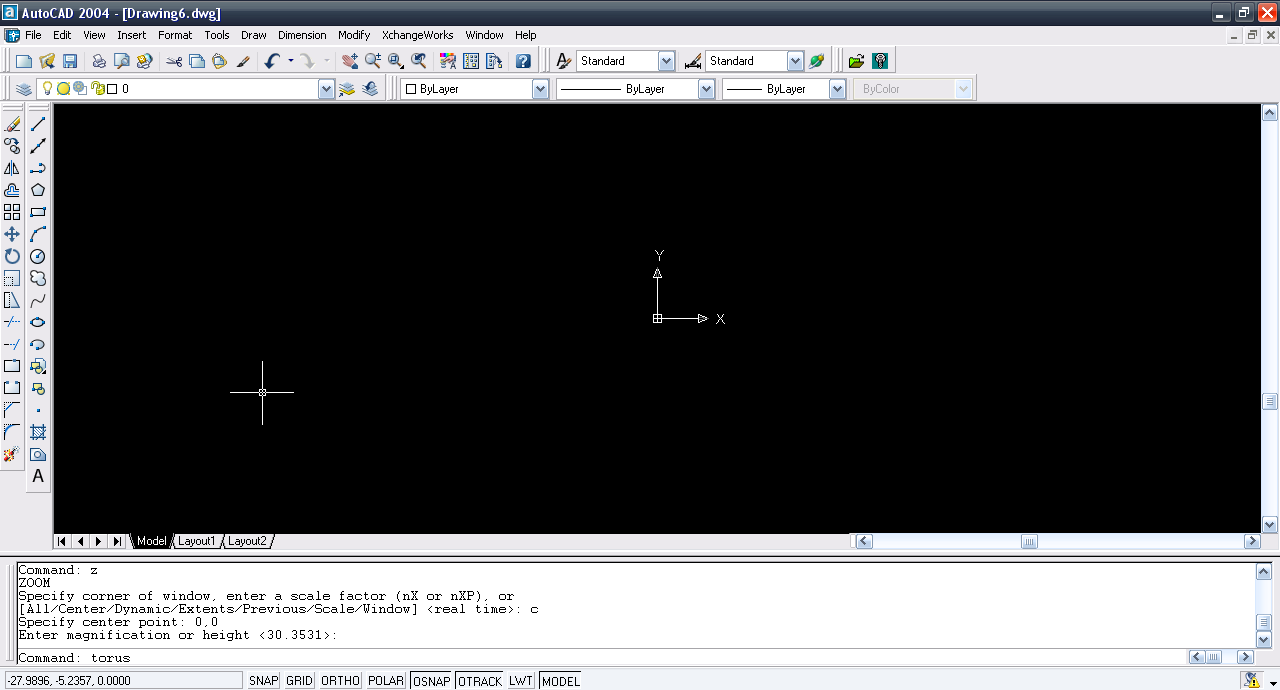2. ### Step 2:

Center at 0,0, Radius of torus = 25, and tube is 2.5.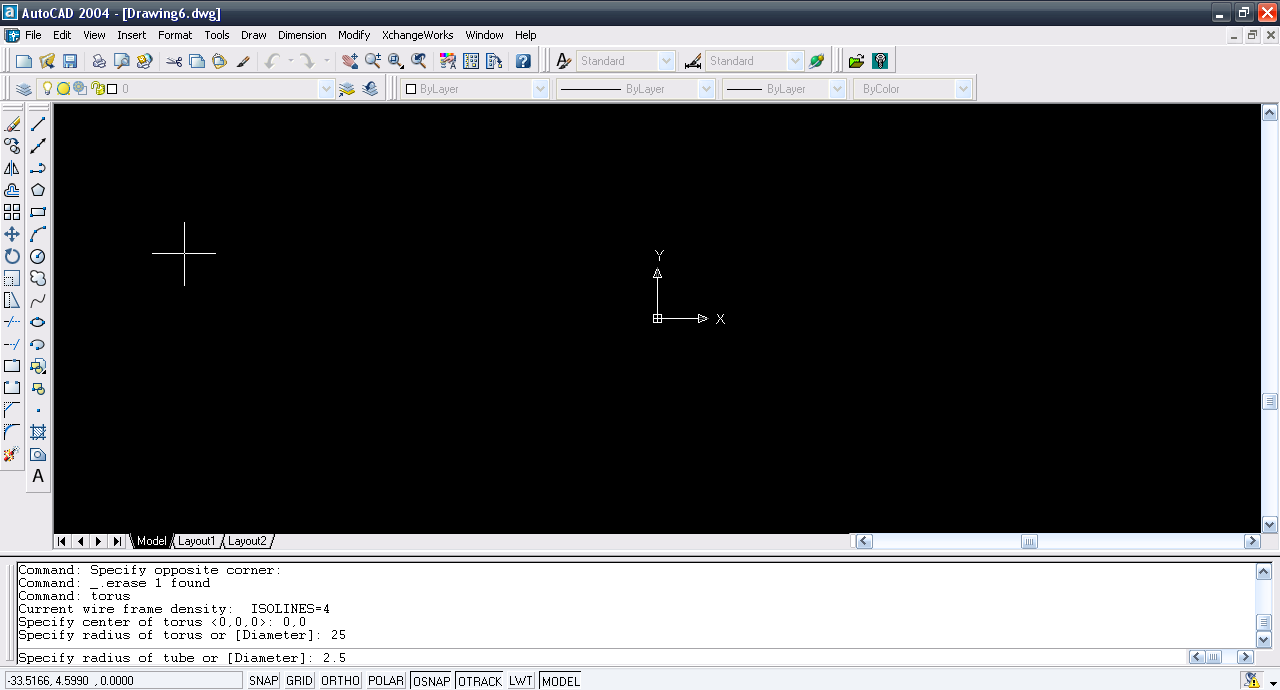3. ### Step 3:

Now we have the torus generated.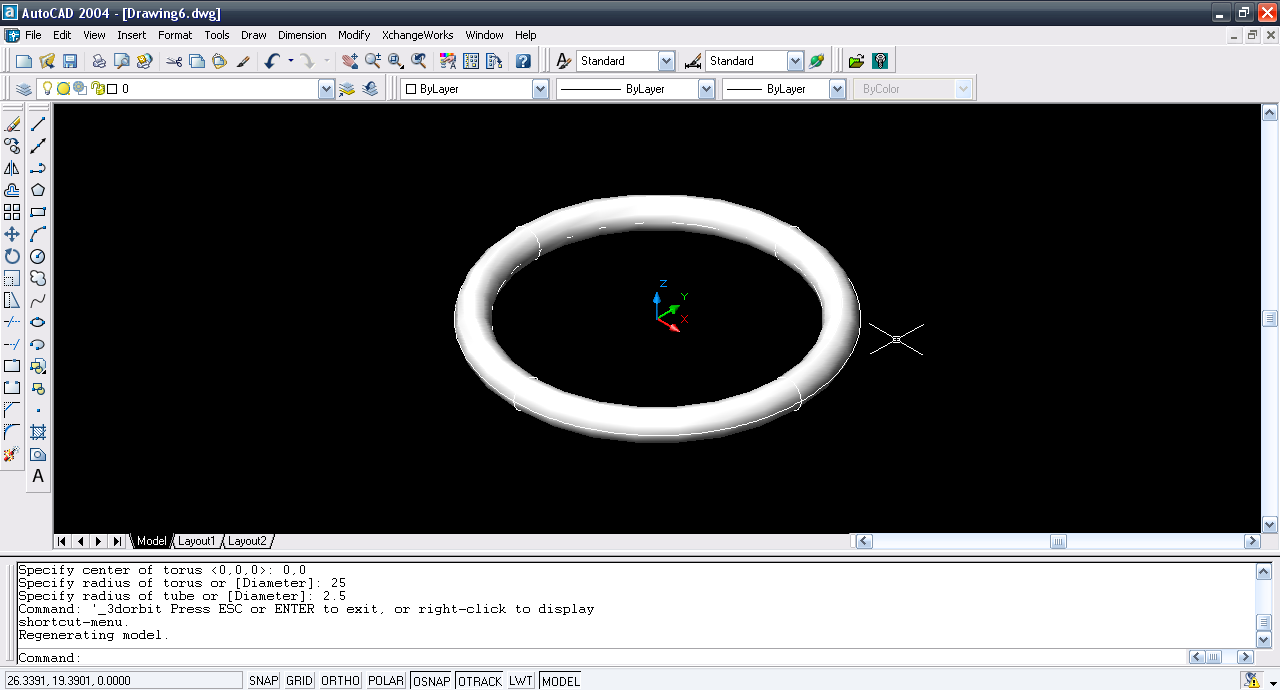4. ### Step 4:

Slice command and select the torus.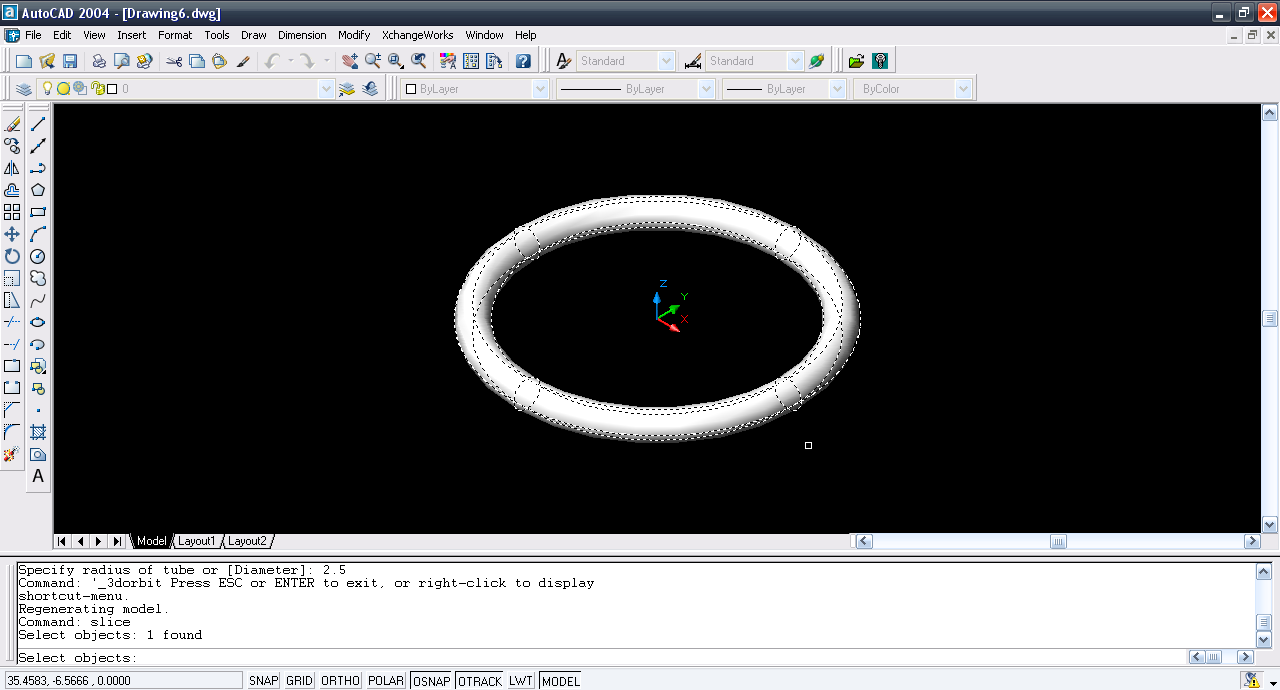5. ### Step 5:

Enter ZX plane.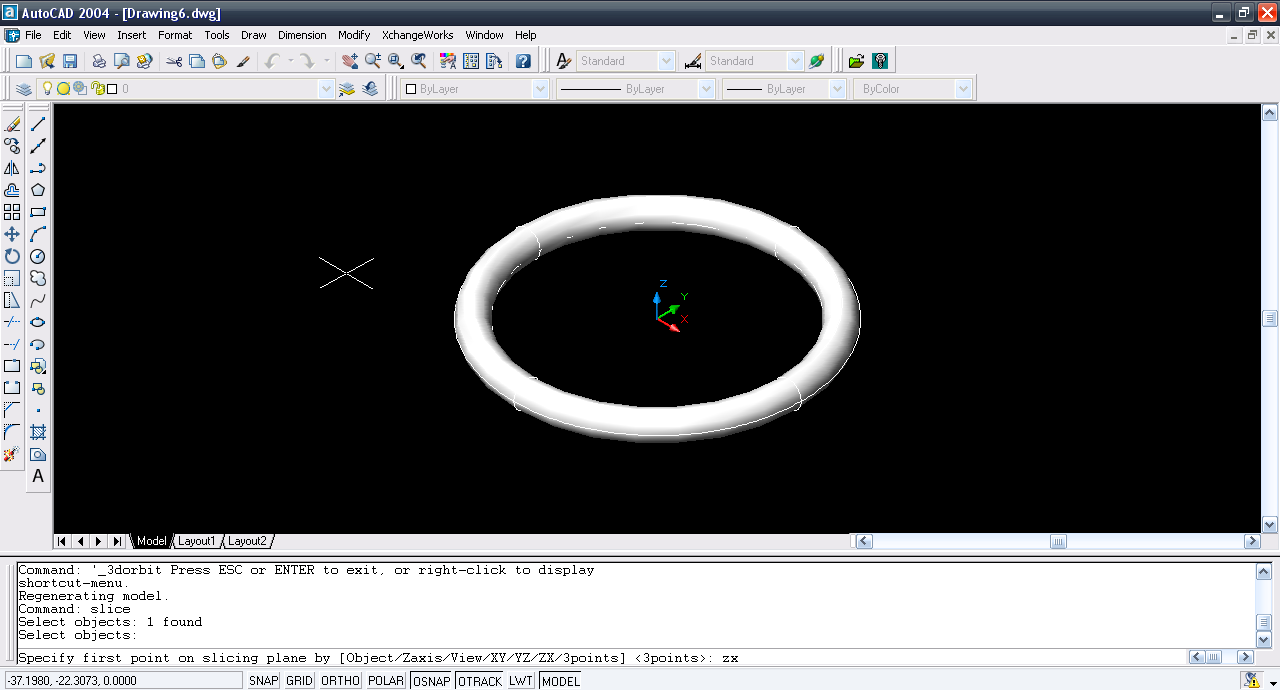6. ### Step 6:

Enter point 0,0,0.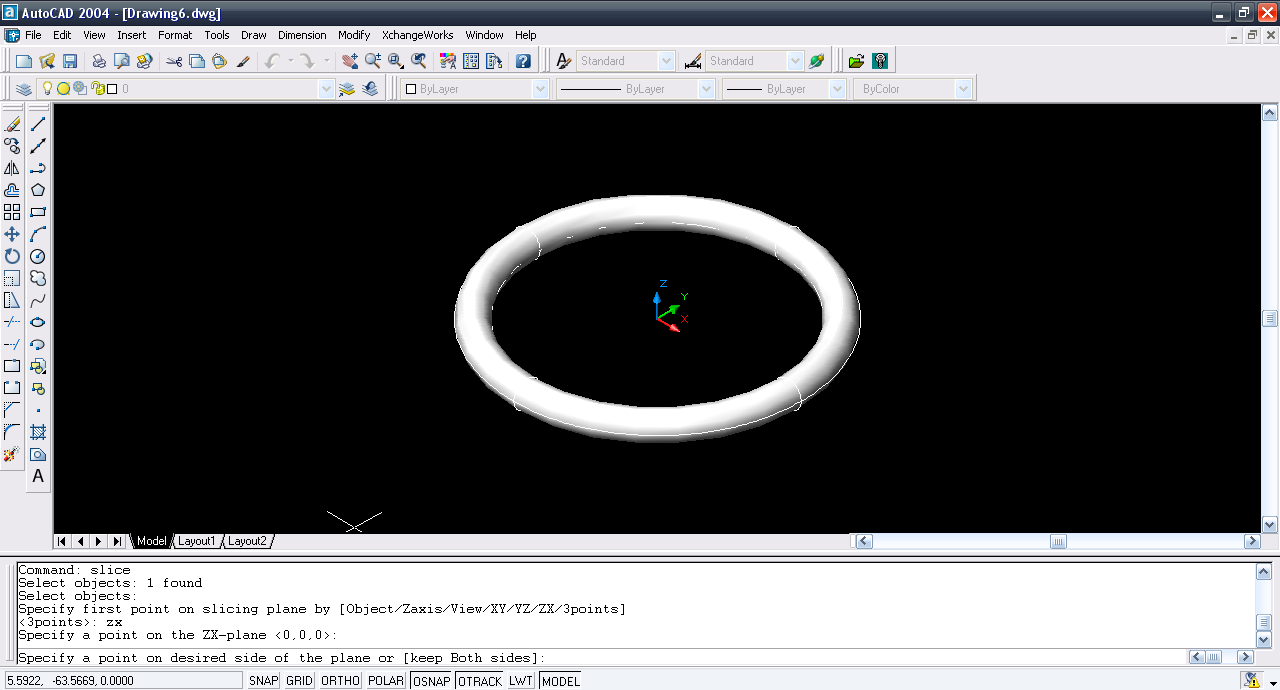7. ### Step 7:

B for both sides.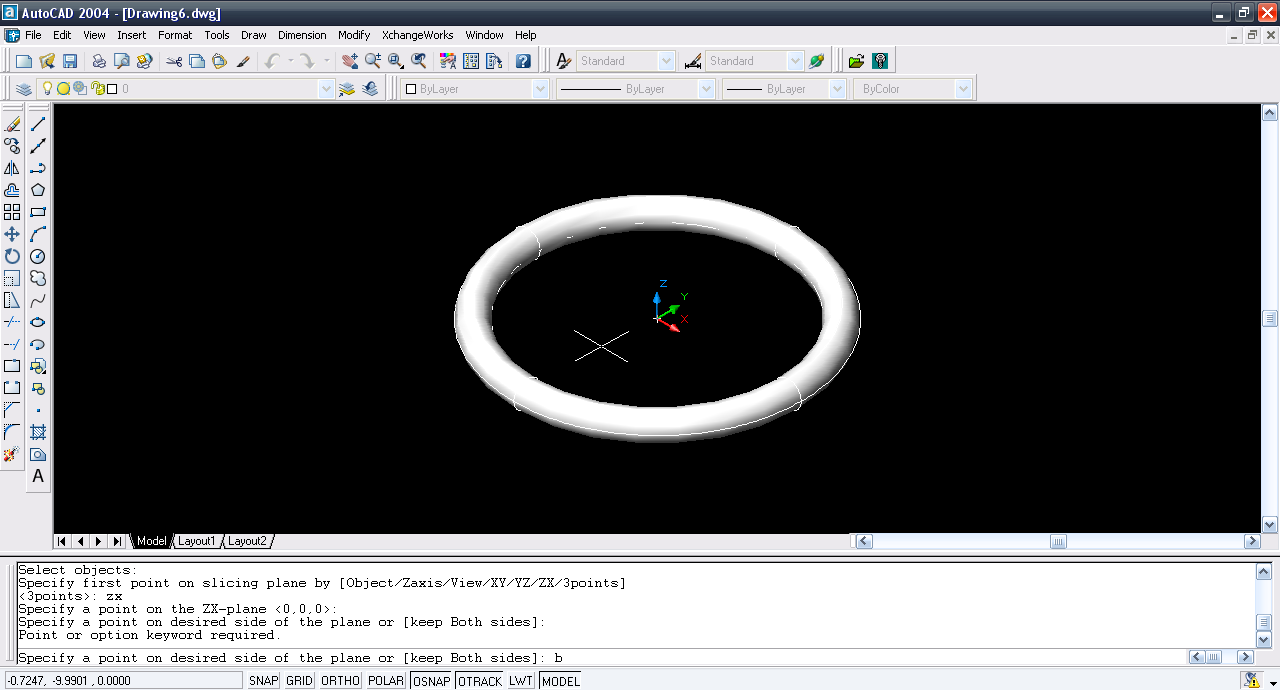8. ### Step 8:

Now enter rotate3d command & select the half of the torus.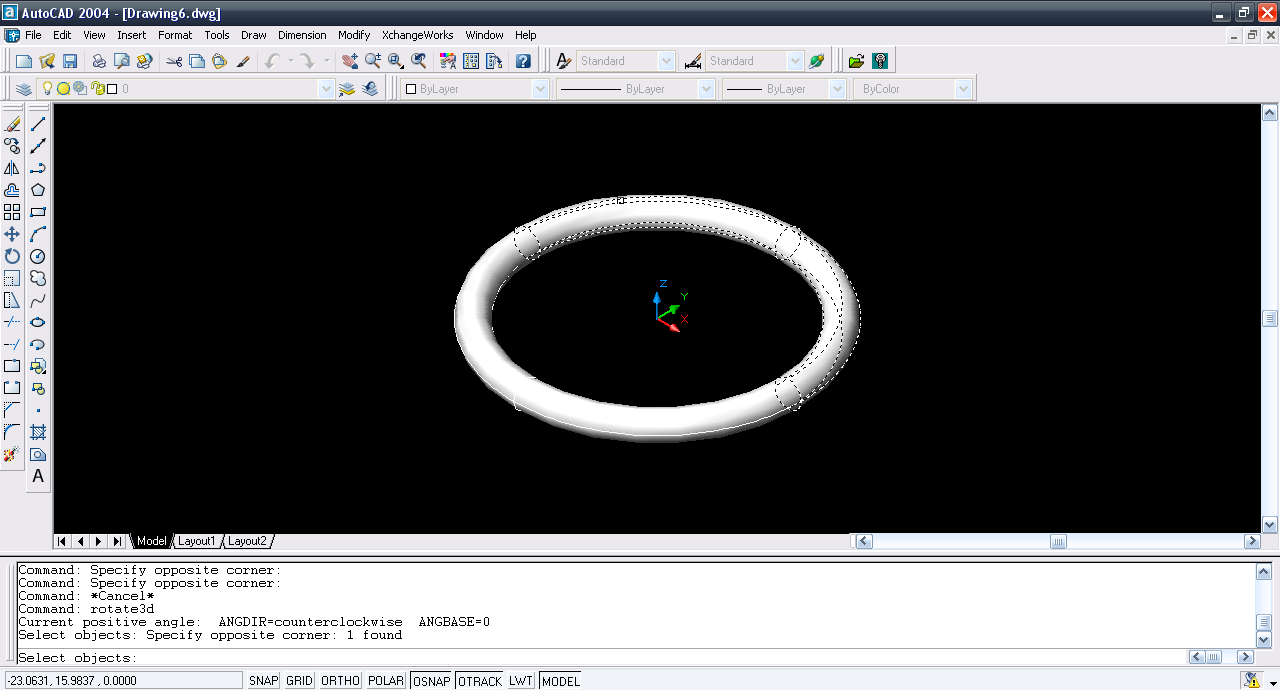9. ### Step 9:

Select Y-axis.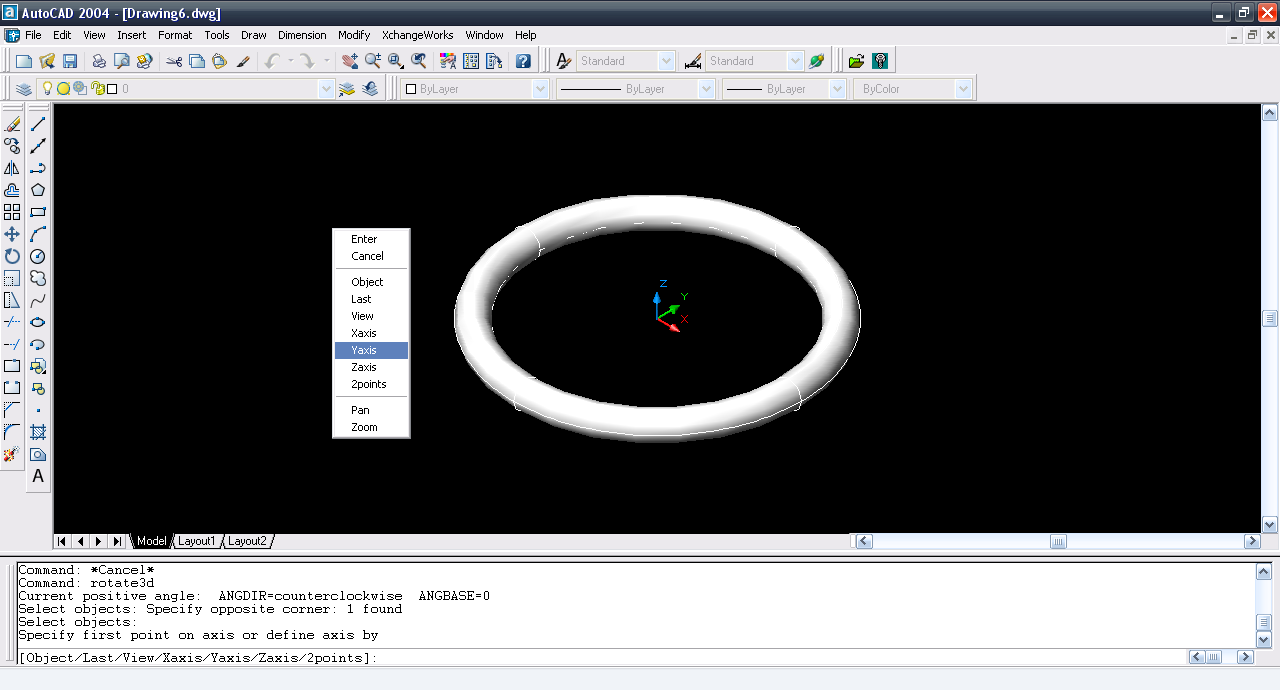10. ### Step 10:

Ctrl+right click and then select center.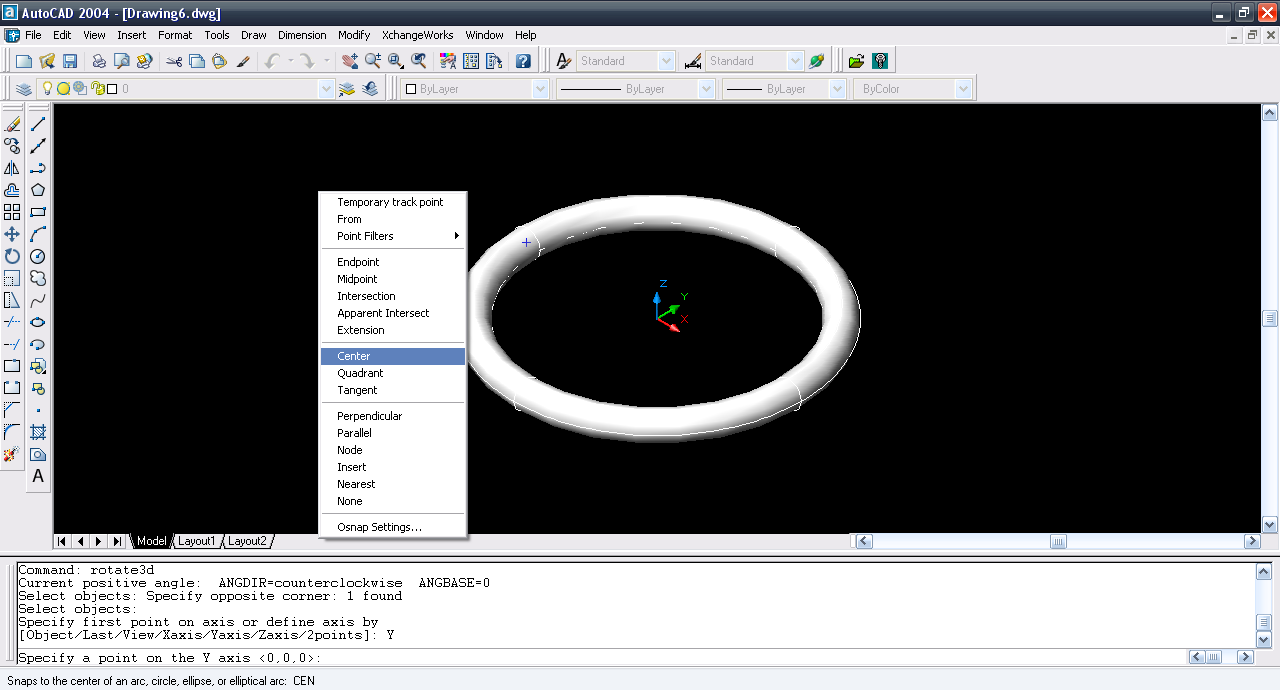11. ### Step 11:

Select the center of the torus.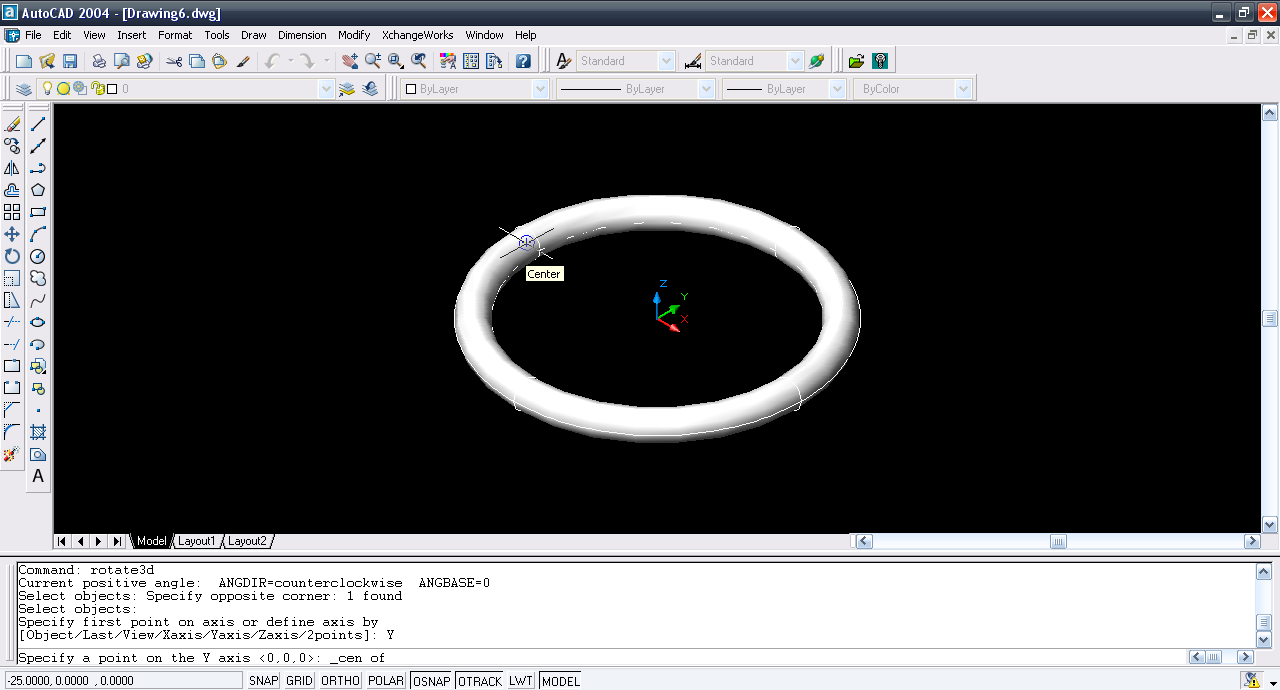12. ### Step 12:

Enter 5º angle.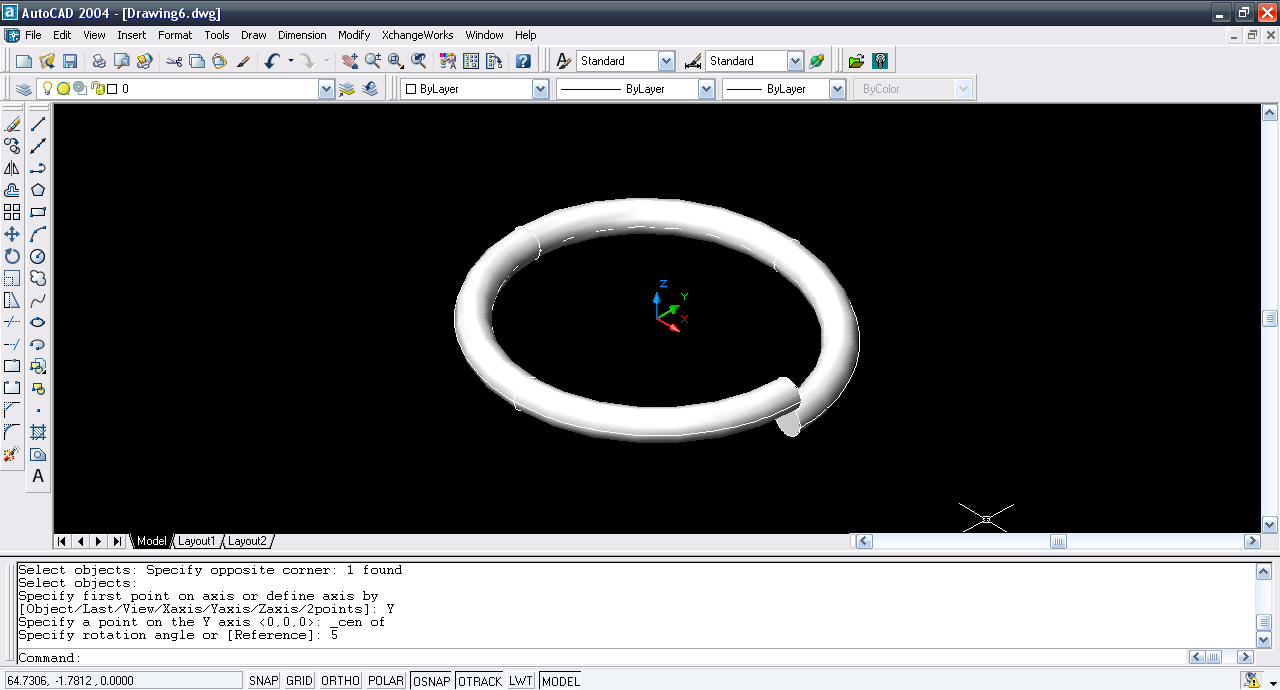13. ### Step 13:

Repeat same step for other half but the angle is -5º.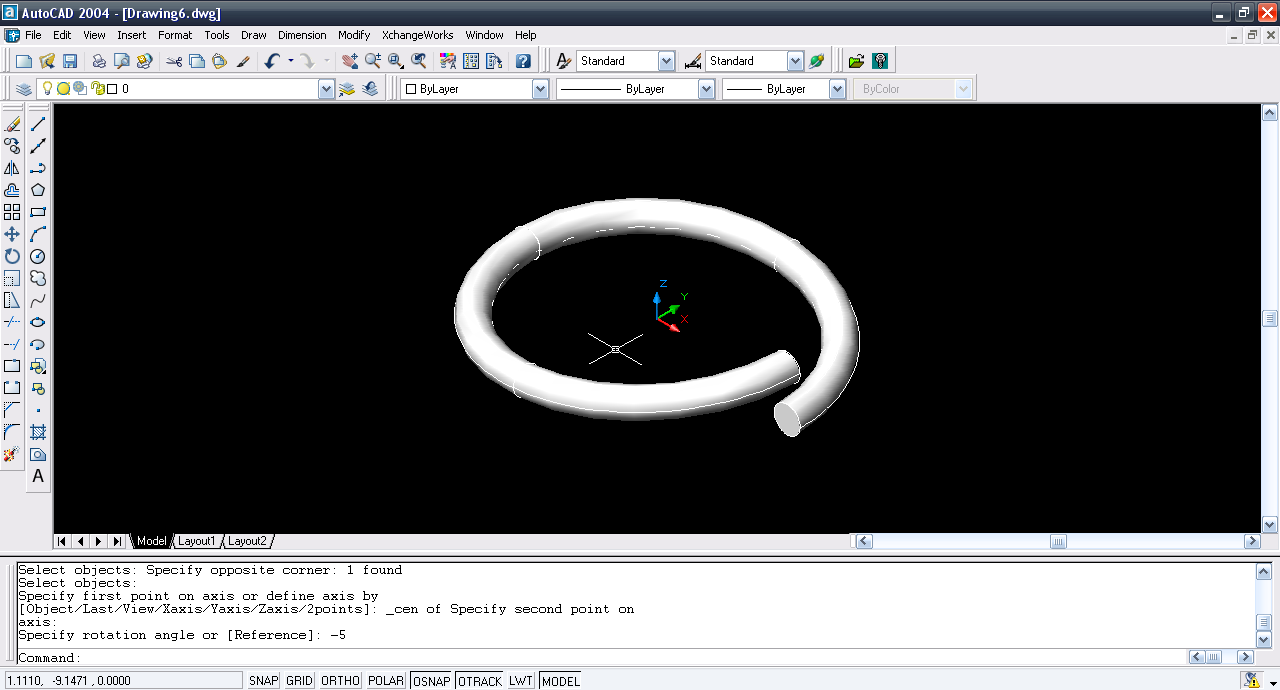14. ### Step 14:

Copy command and select both objects.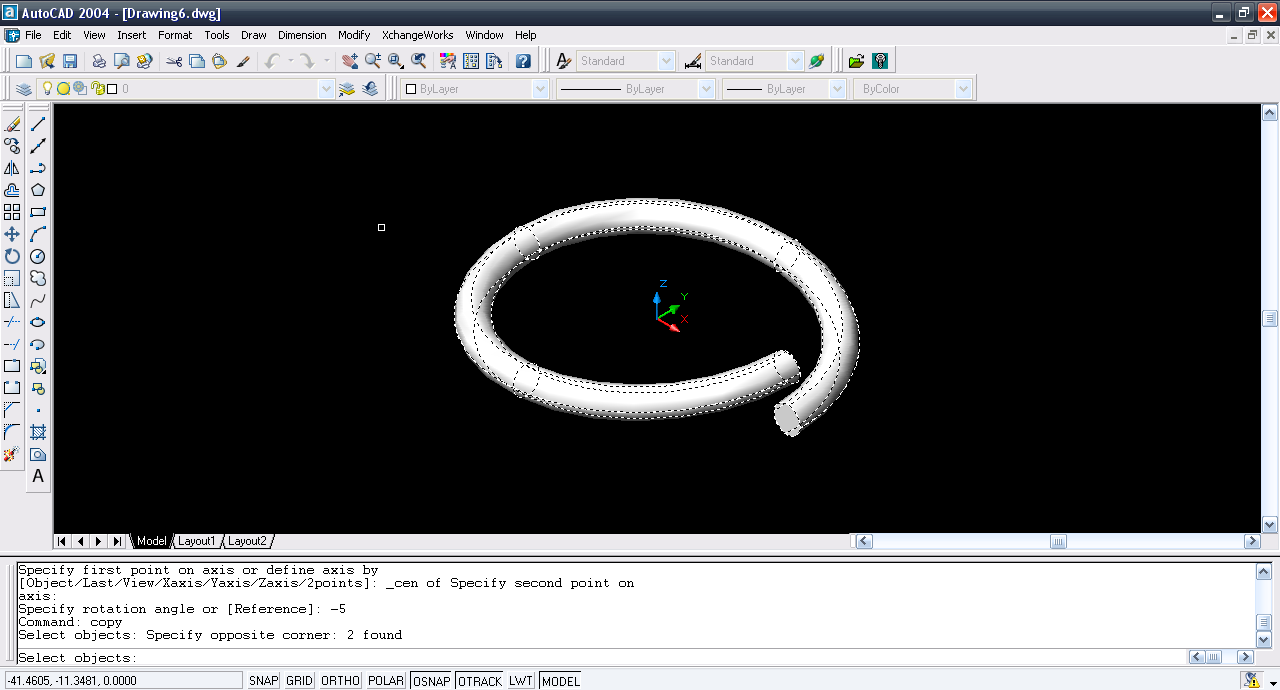15. ### Step 15:

Multiple type.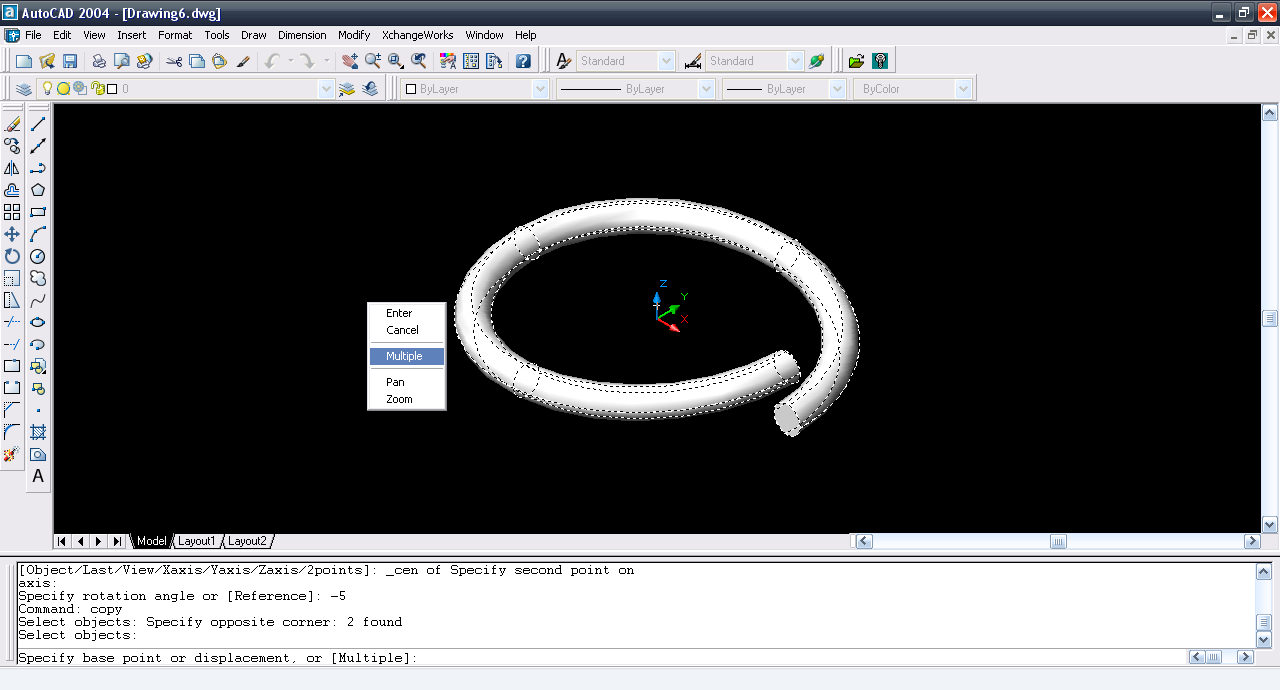16. ### Step 16:

Select the lower point as the base point and the upper point as targer point. Keep repeating it till you get desired spring.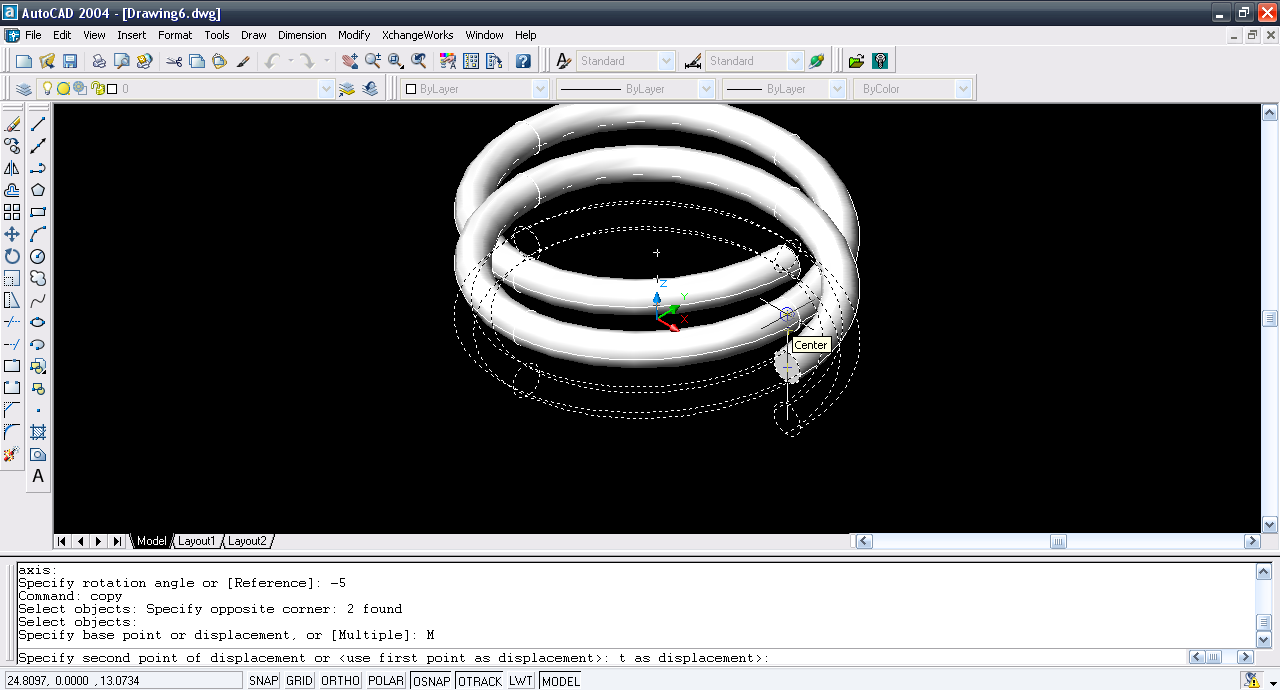17. ### Step 17:

We have the spring.